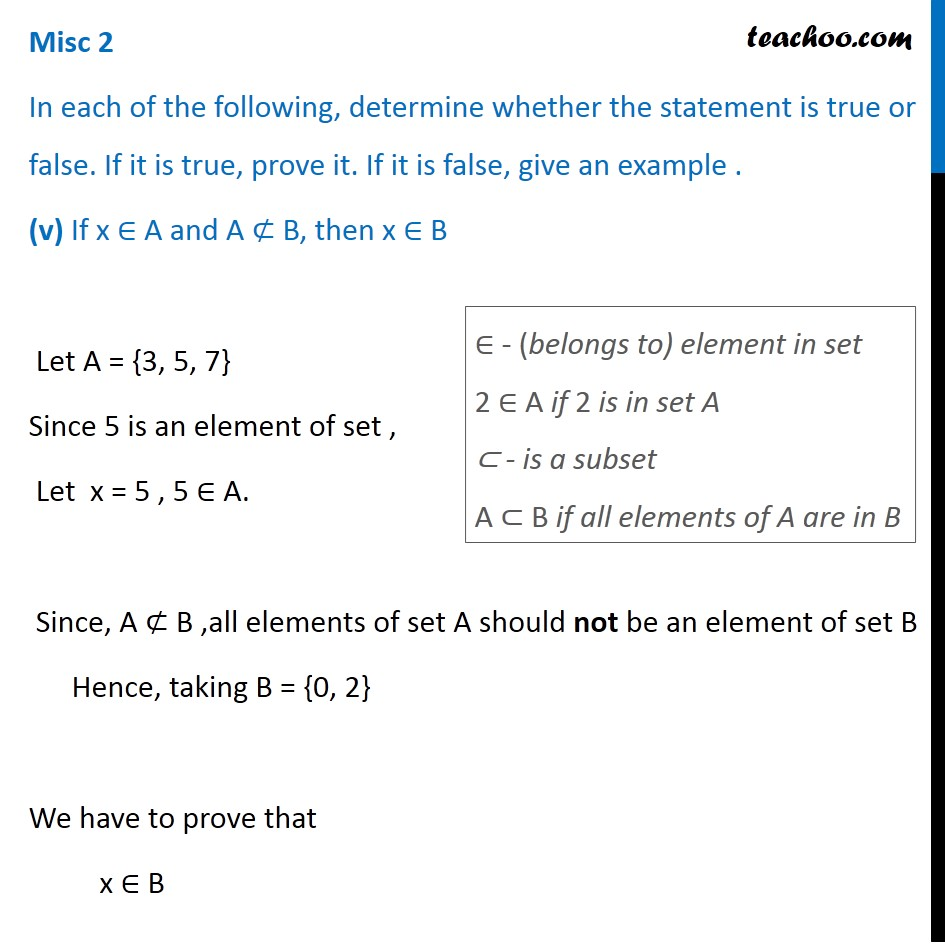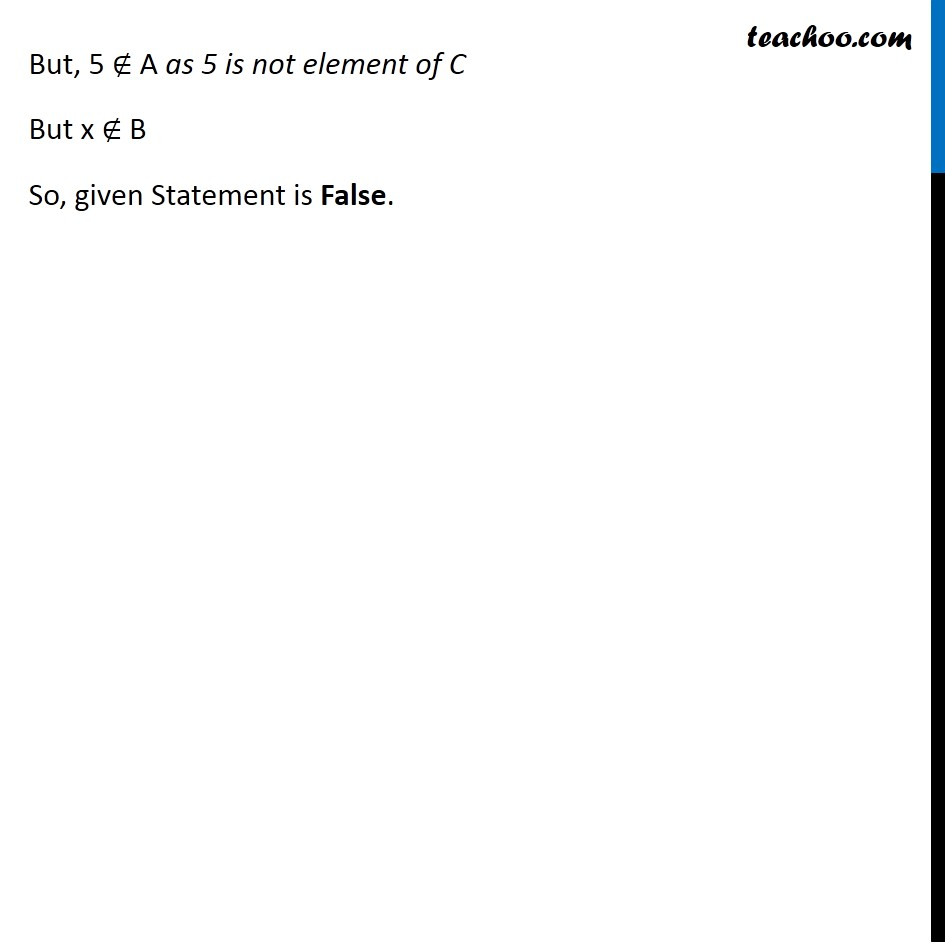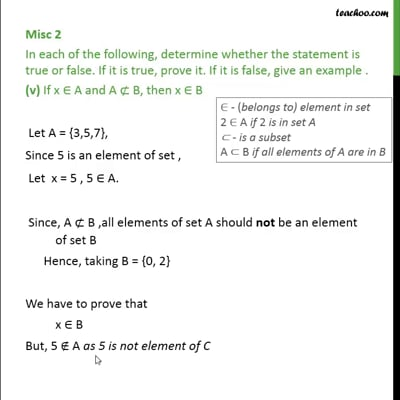Miscellaneous

Chapter 1 Class 11 Sets
Serial order wiseThis video is only available for Teachoo black users

Introducing your new favourite teacher - Teachoo Black, at only ₹83 per month

### Transcript

Misc 2 In each of the following, determine whether the statement is true or false. If it is true, prove it. If it is false, give an example . (v) If x ∈ A and A ⊄ B, then x ∈ B Let A = {3, 5, 7} Since 5 is an element of set , Let x = 5 , 5 ∈ A. Since, A ⊄ B ,all elements of set A should not be an element of set B Hence, taking B = {0, 2} We have to prove that x ∈ B ∈ - (belongs to) element in set 2 ∈ A if 2 is in set A ⊂ - is a subset A ⊂ B if all elements of A are in B But, 5 ∉ A as 5 is not element of C But x ∉ B So, given Statement is False.